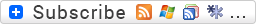# Memorizing the Greeks

by on August 30, 2009

### Memorizing the Greeks

Every experienced option trader should know how to use the option greeks to evaluate their current positions as well as being able to do a “what-if?” analysis to determine what might happen to their positions given changes in price, time, or volatility.  In my experience, most newer traders (and some not-so-new ones) have not taken the time to really understand and memorize the basic, practical rules that affect their ability to analyze their trades.  Not only that, I’ve never seen a concise list of the characteristics I’ve found to be most important.

To that end, I’ve developed a list of what I consider to be the most essential rules and characteristics to remember about the greeks.  Nothing esoteric here, just practical information.

Within each category (greek) I’ve also listed the characteristics from most to least important, and sub-characteristics under each major characteristic as a sub-bullet.  (Tip: if you have trouble remembering the sub-bullets, just memorize the numbered items, since the sub-bullets follow intuitively from concept in the numbered items anyway.)

#### Delta

1. For each one point increase in the underlying price, the value of the delta is added to the value of the option.
• Conversely, for each one point decrease in the price of the underlying, the value of delta is subtracted from the value of the option.
2. The delta of call options is always positive (0 to 100) and the delta of put options is always negative (0 to -100).
3. The delta of an at-the-money option is approximately .50 (calls) or -.50 (puts).
4. The delta of an option at expiration is either 0 or 100 (-100 for puts).

#### Gamma

1. For each one point increase in the underlying price, gamma is added to delta.
• Conversely, for each one point decrease in the price of the underlying, the value of gamma is subtracted from delta.
2. For all positive theta positions, gamma is always negative.
• Conversely, for all negative theta positions, gamma is always positive.
3. For out-of-the-money positions such as condors, gamma is generally small compared to delta and inconsequential, whereas gamma is generally larger and has bigger associated risk for at-the-money positions such as calendars.

#### Theta

1. For each day that passes, the value of theta is added to the value of the option.
2. Long option positions are theta negative (lose time value each day), while short option positions are theta positive (gain time value each day).
3. For longer term options, theta decay is slower, conversely shorter term options have faster theta decay.

#### Vega

1. For every 1% volatility increase in the underlying asset, the value of vega is added to the value of the option.
• Conversely, for every 1% volatility decrease, the value of vega is subtracted from the value of the option.
2. The impact of volatility changes is greater for at-the-money options than it is for in- or out-of-the-money options.
3. The impact of volatility changes is greater for longer term options and less for shorter term options.
4. Changes in vega can have more impact (i.e. you should worry about it more) for multi-month spreads (calendars, diagonals) than for single-month spreads (verticals, condors).

#### Rho

1. For every 1% increase in interest rates, the value of an option increases percentage-wise by the value of rho.
• For example, if the rho of an option is 2.5, and interest rates increase by 1% ,then the value of the option increases by 2.5%.
2. For two reasons, you can usually ignore rho for most practical purposes.  First, interest rates don’t change that often, and second, for short term options, rho is small and doesn’t have much effect.
3. Rho is more important for long term options such as LEAPs.

Jay Bailey
Sheridan Options Mentorcdowis August 30, 2009 at 12:17 pm

I have a trick to remember the importance of delta and gamma, but using a car analogy. Delta is the current speed of the car, and gamma is the acceleration. My delta is now at 30, for example, so I know the risk of my position *right now*, but what happens if there is a move in the market? I may be happy with the risk at 30, but if the gamma is very high, that means that a small movement in the underlying could have explosive results on my risk. Suddenly it goes from 30 to 45, for example.

So, think of delta as current risk (the speed) and gamma as projected risk with a move in the market (acceleration). With market movement, is there just a tap on the accelerator, or is it “pedal to the metal”.Robor September 2, 2009 at 2:10 am

Interesting, I`ll quote it on my site later.
RoborTom Nunamaker September 2, 2009 at 6:52 am

Hi Robor,black hattitude October 16, 2009 at 8:35 pm

hello,

thanks for the great quality of your blog, each time i come here, i’m amazed.Patricia Porter October 9, 2012 at 1:18 am

Some call gamma the delta of the delta.Tom Nunamaker October 9, 2012 at 6:21 am

Gamma is the acceleration of price changes…2nd derivative or the slope of the delta chart. Since delta is the slope of the price chart, that’s an easy way to remember it. Thanks Patricia!

Previous post:

Next post: